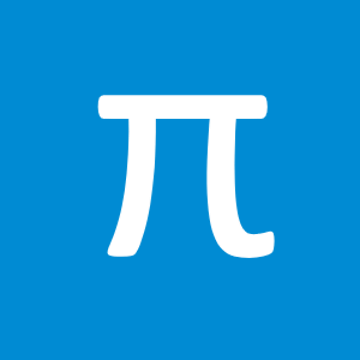FreeOffers in-app purchases
Free+
Overview System Requirements Related

## Description

All you need in math. Step by step solutions and formulas... and many more. - Geometry - Quadratic Function - Linear Function - Math Sequences - Algebra - Vectors - Linear System GEOMETRY - square - rectangle - rhombus - parallelogram - triangle - equilateral triangle - right triangle - isosceles triangle - triangle 30-60-90 - Pythagorean theorem - circle - annulus - trapezoid - right trapezoid - isosceles trapezoid - regular hexagon - sphere - cylinder - cone - regular tetrahedron - cube - square prism - cuboid - ellipse - regular pentagon - kite - square pyramid - triangular pyramid - thales' theorem - triangular prism - regular triangular prism - trigonometry - regular octagon - regular dodecagon - truncated cone - hexagonal prism - pentagonal prism - barrel - truncated square pyramid QUADRATIC FUNCTION - standard form - vertex form - factored form - discriminant of the quadratic function - real roots (zeros) - vertex of a parabola - intersection of the Y-Axis - monotonicity (increasing, decreasing) - positive and negative values (inequalities) LINEAR FUNCTION - slope-intercept form - standard form - distance between two points - midpoint of a line segment - line segment bisector - parallel line - perpendicular line - distance from a point to a line - equation of the line passing through 2 points MATH SEQUENCES - properties of geometric progression: initial term, any mth term and nth term, ratio, sum of n terms, general formula - properties of arithmetic progression: initial term, any mth term and nth term, difference, sum of n terms, general formula - properties of geometric series: initial term, ratio, sum ALGEBRA - greatest common divisor (gcd) - least common multiple (lcm) VECTORS - 2D and 3D - Length of a vector - Dot product - Cross product - Addition and subtraction LINEAR SYSTEM Methods for solving systems: - Substitution method - Elimination method - Method of determinants

## Screenshots

NaNSolvers

NaNSolvers

2/7/2018

1.68 MB

For all ages

#### This app can

Access your Internet connection and act as a server.

#### Installation

Get this app while signed in to your Microsoft account and install on up to ten Windows 10 devices.

#### Accessibility

The product developer believes this product meets accessibility requirements, making it easier for everyone to use.

#### Language supported

English (United States)
Deutsch (Deutschland)
Español (España, Alfabetización Internacional)
Français (France)
Italiano (Italia)
Nederlands (Nederland)
Polski (Polska)
Português (Portugal)
Română (România)
Русский (Россия)
Türkçe (Türkiye)